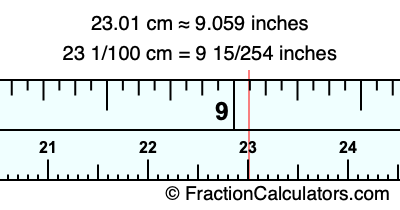23.01 cm in inchesHere is how to convert 23.01 cm to inches. We will give you the fractional answer, the decimal answer, and illustrate the answer on our tape measure.

One centimeter is equal to 50/127 inches, and 23.01 as a fraction is 23 1/100. Therefore, to get the fractional answer to 23.01 cm in inches, we multiply 23 1/100 by 50/127 and simplify if necessary.

cm × 50/127 = inches
23 1/100 × 50/127 = inches
23 1/100 × 50/127 = 9 15/254
23 1/100 cm = 9 15/254 inches
23.01 cm = 9 15/254 inches

As promised above, we also have the answer to 23.01 cm in inches in decimal form. Although the fractional answer above is exact, the decimal answer may be rounded if necessary:

9 15/254 ≈ 9.05905511811024
9 15/254 ≈ 9.059
23.01 cm ≈ 9.059 inches

Our image below shows 23.01 cm on a tape measure. The top row of the tape measure is inches, and the bottom row is centimeters.As you can see, we drew a red line where 23.01 cm and 9.059 inches meet on the measuring tape.

Centimeters to Inches Calculator
Here you can convert another length in centimeters to inches.

Convert  cm to inches.

What is 23.02 cm in inches?
Here is another inches to centimeters calculation we did for you.